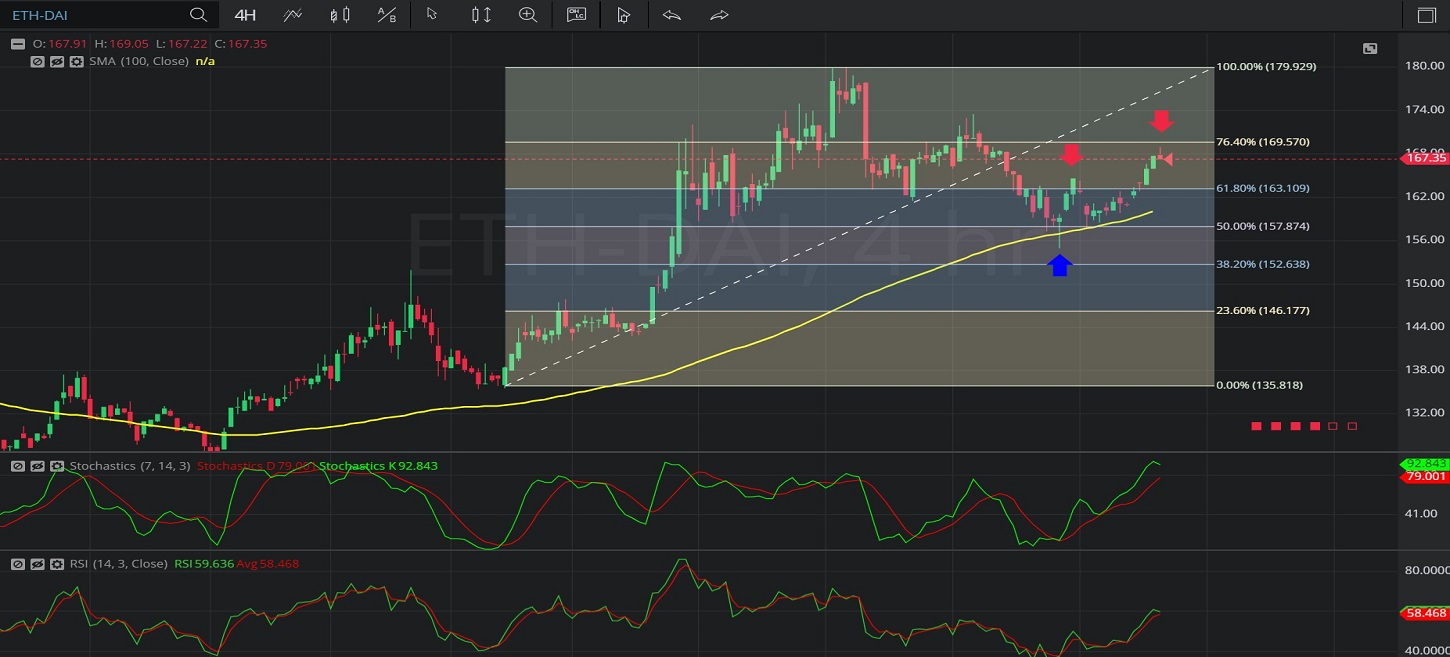Hot Topics

## 28 January 2020 - ETH/ DAI – 4-hourlyIn this 4-hourly chart of Ethereum / Multi-collateral DAI (ETH/ DAI), there is an appreciating range from 135.48 to 180.00.

We can observe that the market then depreciated to the 155.02 level (identified by the blue up arrow), below the 157.74 area that represents the 50% retracement of the appreciating range.

We can observe that this 155.02 area was also around the 100-bar Simple Moving Average (identified in yellow).

We can observe that the pair then appreciated to the 164.62 level (identified by the first red down arrow), just above the 169.49 area that represents the 23.6% retracement of the appreciating range.

We can observe that the during the pair’s move higher, bids emerged around the 157.74 area.

We can observe that the market continues to appreciate (identified by the second red down arrow).

Using RSI, we can observe that the slope of RSI (14) and the slope of RSI Average (3) turned positive after the market depreciated to the 155.02 area, and that RSI (14) bullishly crossed above RSI Average (3).  We can observe that RSI (14) is currently indicating above RSI Average (3).

Using Slow Stochastics, we can observe that the slope of Stochastics K and the slope of Stochastics D turned positive after the market depreciated to the 155.02 area, and that Stochastics K bullishly crossed above Stochastics D.  We can observe that Stochastics K is currently indicating above Stochastics D.

Stay on top of the cryptocurrency market with CryptoDaily’s trading charts!

https://charts.cryptodaily.net/